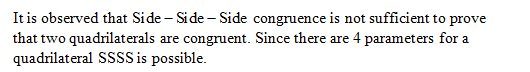# Is there an analog to the SSS triangle congruence theorem for quadrilaterals?

Question
73 views

Is there an analog to the SSS triangle congruence theorem for quadrilaterals?

check_circle

Step 1

To show whether there is an analog to the SSS triangle congruence theorem for quadrilateral.

Step 2

The SSS triangle congruence theorem states that if all 3 sides of one triangle are in proportion to all 3 sides of another triangle then those triangles are similar.

Step 3...

### Want to see the full answer?

See Solution

#### Want to see this answer and more?

Solutions are written by subject experts who are available 24/7. Questions are typically answered within 1 hour.*

See Solution
*Response times may vary by subject and question.
Tagged in

### Math